#### Chapter 8 Lines and Angle R.D. Sharma Solutions for Class 9th Math Exercise 8.2

Exercise 8.2

1. In Fig. 8.31, OA and OB are opposite rays:(i) If x = 25°, what is the value of y?
(ii) If y = 35°, what is the value of x?

Solution2 . In Fig. 8.32, write all pairs of adjacent angles and all the linear pairs.Solution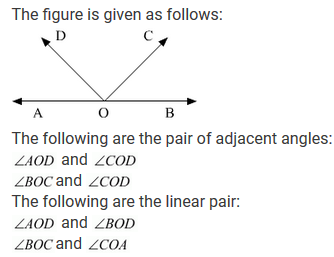3.In Fig. 8.33, find x. Further find ∠BOC, ∠COD and∠AOD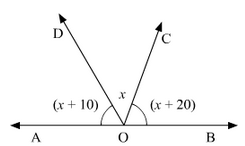Solution

Since,
∠AOD and ∠BOD from a linear pair.
∠AOD + ∠BOD = 180°
∠AOD + ∠BOC+ ∠COD = 180°
Given that,
∠AOD = (x+10°),
∠COD = x° and,
∠BOC = (x+20)°
(x+10) + x + (x+20) = 180°
⇒ 3x + 30 = 180
⇒ 3x = 180 - 30
⇒ 3x = 180 - 30
⇒ 3x = 150
⇒ 3x = 150/3
⇒ x = 50
Therefore,
∠AOD = (x+10°) = 50+10 = 60
∠COD = x = 50
∠BOC = x+20 = 50+20 = 70
Thus, AOD = 60°, ∠COD = 50° and ∠BOC = 70°

4. In Fig. 8.34, rays OA, OB, OC, OD and OE have the common end point O. Show that ∠AOB + ∠BOC + ∠COD + ∠DOE + ∠EOA = 360°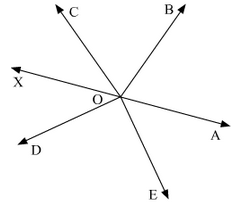Solution
Let us draw a straight line, AOX.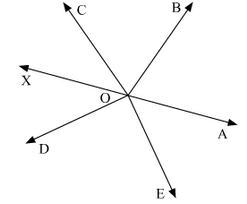5. In the figure given below, it is given that ∠AOC and ∠BOC forms a linear pair.

Solution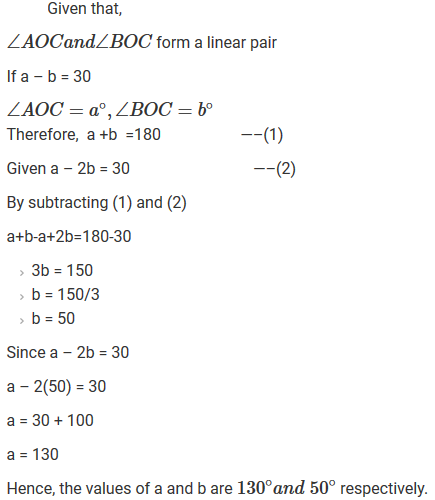6. How many pairs of adjacent angles are formed when two lines intersect at a point ?

Solution
Four pairs of adjacent angles will be formed when two lines intersect at a point.
Considering two lines AB and CD intersecting at O
The 4 pairs are :
(i) ∠AOC = ∠BOC
(ii) ∠BOC = ∠DOB
(iii) ∠AOC = ∠AOD
(iv) ∠DOB = ∠AOD

7. How many pairs of adjacent angles, in all, can you name in Fig. 8.36.Solution

In the given figure,We have 10 adjacent angle pairs, namely:
(i) ∠AOB and ∠BOC
(ii) ∠AOB and ∠BOD
(iii) ∠AOB and ∠BOE
(iv) ∠BOC and ∠COD
(v) ∠BOC and ∠COE
(vi) ∠COD and ∠DOE
(vii) ∠COD and ∠AOC
(viii) ∠COD and ∠BOC
(ix) ∠AOC and ∠COE
(x) ∠AOD and ∠DOE

8.  In Fig. 8.37, determine the value of x.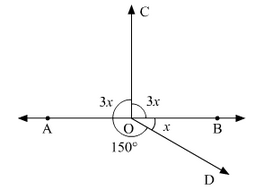Solution

In the given figure:Since, the sum of all the angles round a point is equal to 360°.
3x+3x+150+x = 360°
⇒ 7x = 360 - 150
⇒ 7x = 210
⇒ x = 210/7
⇒ x = 30
Value of x is 30°.

9. In Fig. 8.38, AOC is a line, find x.

Solution

Since,
∠AOB and ∠BOC are linear pairs,
∠AOB and ∠BOC = 180°
70+2x = 180°
⇒ 2x = 180°-70°
⇒ 2x = 180°-70°
⇒ x = 110°/2
⇒ x = 55°

10. In Fig. 8.39, POS is a line, find x.Solution

The figure is given as follows:Since ∠POQand∠QOS are linear pairs
∠POQ+∠QOS=180∘
∠POQ+∠QOR+∠SOR=180∘
60 + 4x +40 = 180
⇒ 4x = 180 -100
⇒ 4x = 80
⇒ x = 20
Hence, Value of x = 20

11. In Fig. 8.40, ACB is a line such that ∠DCA = 5x and ∠DCB = 4x. Find the value of x.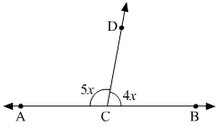Solution

It is given that ACB is a line in the figure given below.
Thus, ∠ACD and ∠BCDform a linear pair.Therefore, their sum must be equal to 180°.
Or, we can say that
∠ACD + ∠BCD = 180°
Also, ∠ACD = 4x and ∠BCD = 5x
This is simplified as,
4x + 5x = 180°
⇒ 9x = 180°
⇒ x = 180/9
⇒ x = 20
Hence, the value of x is 20°.

12. Given ∠POR = 3x and ∠QOR = 2x + 10, find the value of x for which POQ will be a line. (Fig. 8.41).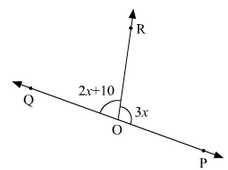Solution13. In Fig. 8.42, a is greater than b by one third of a right-angle. Find the values of a and b.Solution
Since a and b are linear
a + b = 180
⇒ a = 180 – b —(1)

From given data, a is greater than b by one third of a right angle
a = b + 90/3
⇒ a = b + 30
⇒ a – b = 30  —(2)

Equating (1) and (2),
180 – b = b + 30
⇒ 180 – 30 = 2b
⇒ b = 150 / 2
⇒ b = 75

From (1)
a = 180 – b
⇒ a = 180 – 75
⇒ a = 105
Hence, the values of a and b are 105° and 75° respectively .

14. What value of y would make AOB a line in Fig. 8.43, if ∠AOC = 4y and ∠BOC = (6y + 30)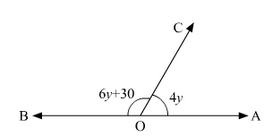SolutionSince, ∠AOCand∠BOC are linear pairs ∠AOC+∠BOC=180°
6y + 30 + 4y = 180
⇒ 10y + 30 = 180
⇒ 10y = 180 – 30
⇒ 10y = 150
⇒ y = 150/10
⇒ y = 15

15. If Fig. 8.44, ∠AOF and ∠FOG form a linear pair.∠EOB = ∠FOC = 90° and ∠DOC = ∠FOG = ∠AOB = 30°.(i) Find the measure of ∠FOE, ∠COB and ∠DOE.
(ii) Name all the right angles.
(iii) Name three pairs of adjacent complementary angles.
(iv) Name three pairs of adjacent supplementary angles.
(v) Name three pairs of adjacent angles.

Solution

The given figure is as follows:(ii) Right angles are ∠DOG,∠COF,∠BOF,∠AOD
(iii) Adjacent complementary angles are (∠AOB,∠BOD);(∠AOC,∠COD);(∠BOC,∠COE);
(iv) Adjacent supplementary angles are (∠AOB,∠BOG);(∠AOC,∠COG);(∠AOD,∠DOG);
(v) Adjacent angles are (∠BOC, ∠COD);(∠COD,∠DOE);(∠DOE,∠EOF);

16 . In Fig. 8.45, OP, OQ, OR and OS  are four rays. Prove that:∠POQ + ∠QOR + ∠SOR + ∠POS = 360° .Solution17. In Fig. 8.46, ray OS stand on a line POQ, Ray OR and ray OT are angle bisectors of ∠POS and∠SOQ respectively. If ∠POS = x, find ∠ROT.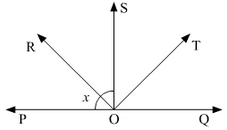Solution18. In Fig, 8.47, lines PQ and RS intersect each other at point O. If ∠POR: ∠ROQ = 5 : 7, find all the angles.Solution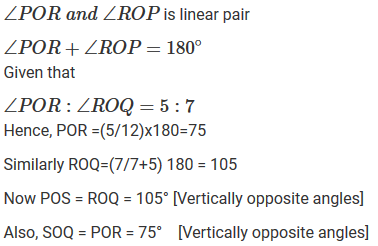19. In Fig. 8.48, POQ is a line. Ray OR is perpendicular to line PQ. OS is another ray lying between OP and OR. Prove that ∠ROS = 1/2 (∠QOS - POS)

SolutionGiven that,
OR is perpendicular to line PQ
To prove,
∠ROS = 1/2(∠QOS – ∠POS)
A/q,
∠POR = ∠ROQ = 90° (Perpendicular)
∠QOS = ∠ROQ + ∠ROS = 90° + ∠ROS --- (i)
∠POS = ∠POR - ∠ROS = 90° - ∠ROS --- (ii)
Subtracting (ii) from (i)
∠QOS - ∠POS = 90° + ∠ROS - (90° - ∠ROS)
⇒ ∠QOS - ∠POS = 90° + ∠ROS - 90° + ∠ROS
⇒ ∠QOS - ∠POS = 2∠ROS
⇒ ∠ROS = 1/2(∠QOS – ∠POS)
Hence, Proved.